Get our FREE VBA eBook of the 30 most useful Excel VBA macros.

Automate Excel so that you can save time and stop doing the jobs a trained monkey could do.

# Spreadsheet Day 2018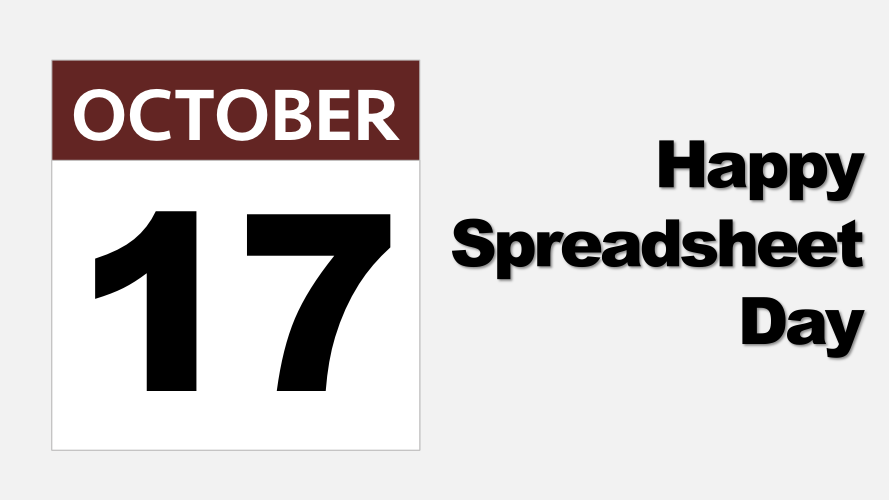17th of October is Spreadsheet Day, the day each year when we celebrate… you guessed it… spreadsheets.

VisiCalc, the first spreadsheet application, shipped on 17 October 1979. Originally it was only compatible with the Apple II and cost \$99. I don’t know if VisiCalc had formulas and functions. Sometimes I wonder what the first spreadsheet function was? I’m guessing it was SUM, surely that would be the most obvious place to start.

So to celebrate Spreadsheet Day, I wanted to write a post about the SUM function and discover some of the tricks which the earliest spreadsheet users may have used to give it more power.

In this post, there are lots of array formulas. These can be identified as those with curly brackets ( { } ) at the start and finish of the formula. Do not enter the curly brackets ( { } ), Excel will add these itself when pressing Ctrl + Shift + Enter to enter the formula.

## Basic SUM Function

In this first example, we have the SUM function as we all know it. Nothing fancy or complicated going on here.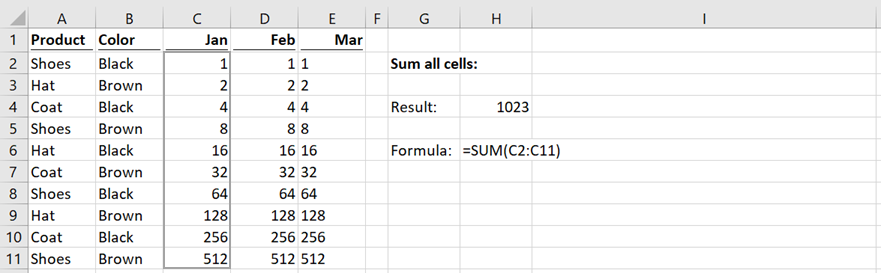The formula in cell H4 is:

`=SUM(C2:C11)`

This formula will sum all the values from Cells C2 – C11. Simple.

## SUM non-contiguous range

SUM does not have to be used on a single range; they can also be disconnected ranges as shown in the example below. The comma ( , ) between the ranges is called the Union operator and is used to join ranges together.The formula in cell H4 is:

`=SUM(C2:C5,C10,D7:D8,D10:D11)`

This formula will sum all the ranges listed as if they were within a single range.

## SUM intersection between two ranges

A space between two ranges is known as the Intersection Operator (a fancy way of describing the cells contained in two ranges which cross-over).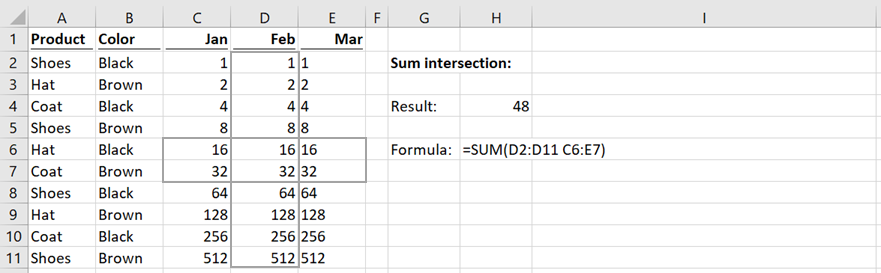The formula in cell H4 is:

`=SUM(D2:D11 C6:E7)`

This formula will sum the cells D6-D7, as these are the cells contained in both D2-D11 and C6-E7).

## SUM numbers formatted as text

When numbers are formatted as text, it causes issues for the SUM function. But we can get around it with a bit of formula magic.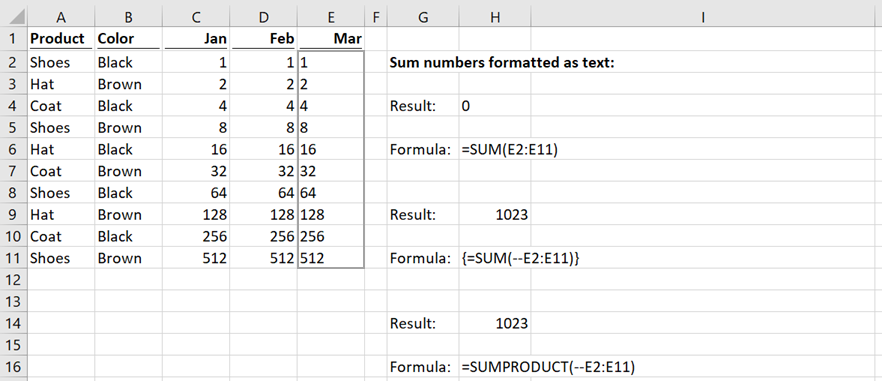In the screenshot above the cells in E2-E11 are all preceded by a single quotation mark ( ‘ ), therefore Excel will treat them as text.

The formula in cell H4 is:

`=SUM(E2:E11)`

The result of this is zero because these are not numbers, but text. Which is a problem if we actually want to sum the values.

When a number is formatted as text, multiplying it by 1 or by — (minus minus) will convert it into a number.

The formula in cell H9 is:

`{=SUM(--E2:E11)}`

This is an array formula, which converts the text to numbers, then adds the result.

The formula in cell H14 is:

`=SUMPRODUCT(--E2:E11)`

This is a method to calculate the same result without using an array formula.

## SUM if with single criteria

SUMIF is a common formula used to add cells where a single condition is met. But SUM can achieve this by itself.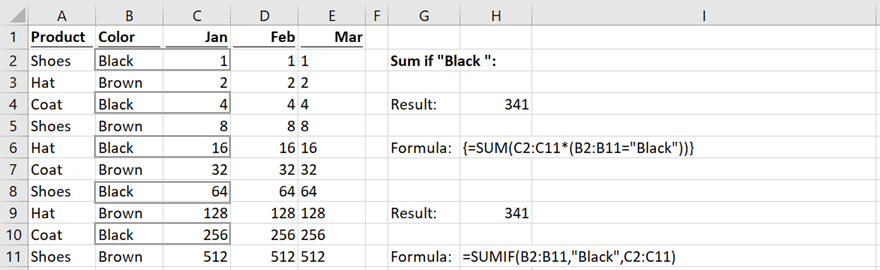The formula in cell H4 is:

`{=SUM(C2:C11*(B2:B11="Black"))}`

This array formula will sum the values in C2-C11 where Cells B2-B11 is equal to the text “Black”.

The formula in cell H14 is:

`=SUMIF(B2:B11,"Black",C2:C11)`

Using SUMIF will achieve the same result without using an array formula.

## SUM if greater than

Following on from the previous example, we can also use SUM to only include the values over a specific amount.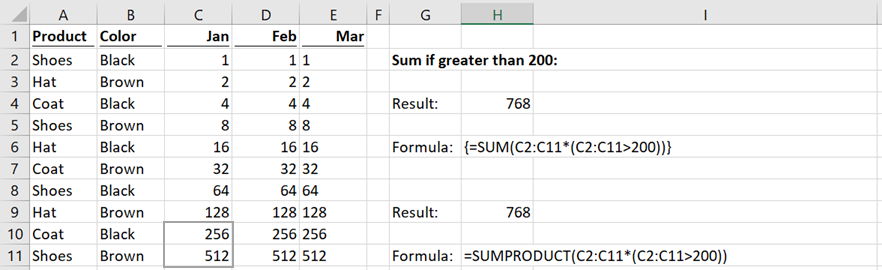The formula in cell H4 is:

`{=SUM(C2:C11*(C2:C11>200))}`

This array formula will SUM only the values which are greater than 200.

The formula in cell H9 is:

`=SUMPRODUCT(C2:C11*(C2:C11>200))`

By changing SUM for SUMPRODUCT, we can achieve the same result but without the need to press Ctrl + Shift + Enter. SUMPRODUCT is a function which can handle arrays, which makes it a powerful formula.

## SUM if with multiple criteria

When using multiple criteria, SUMIF doesn’t work. Pre-Excel 2007, SUMPRODUCT would have been the option many users would have gone for. In Excel 2007 the SUMIFS function was introduced, which allowed multiple conditions to be used. But wait… the SUM function can also sum based on multiple conditions.The formula in Cell H4 is:

`{=SUM(C2:C11*(B2:B11="Black")*(A2:A11="Shoes"))}`

This array formula will SUM only the cells in Cells C2-C11 where B2-B11 is equal to “Black” and Cells A2-A11 is equal to “Shoes”.

The formula in Cell H9 is:

`=SUMIFS(C2:C11,B2:B11,"Black",A2:A11,"Shoes")`

SUMIFS is a non-array formula which faster and easier to understand than using the SUM array formula.

## SUM across two dimensions

SUM is also happy working with criteria across both rows and columns.The formula in Cell H4 is:

`{=SUM(C2:D11*(B2:B11="Black")*(C1:D1="Feb"))}`

This array formula will sum the records which meet the criteria of “Black” from the rows and “Feb” from the columns.

The formula in Cell H9 is:

`=SUMPRODUCT((C2:D11)*(B2:B11="Black")*(C1:D1="Feb"))`

SUMPRODUCT is a more convenient way of dealing with this type of scenario.

## SUM largest values

By combining the LARGE function with the SUM function, it is possible to sum the largest values. Within our example, the largest cells are grouped at the bottom, but this works equally well when the values are spread over the data set.The formula in Cell H4 is:

`{=SUM(LARGE(C2:C11,{1,2,3,4,5}))}`

This is an array formula which will sum the five largest values. To amend this, change the list within the curly brackets at the end. e.g. change {1,2,3,4,5} to {1,3,5} to sum the 1st, 3rd and 5th largest values.

The formula in Cell H9 is:

`=SUM(AGGREGATE(14,4,C2:C11,{1,2,3,4,5}))`

This formula uses the AGGREGATE function to avoid the array function. Just like SUMPRODUCT, AGGREGATE can handly arrays natively without the need for Ctrl + Shift + Enter.

## SUM smallest values

The SMALL and LARGE functions are very similar in their application. As shown by this example.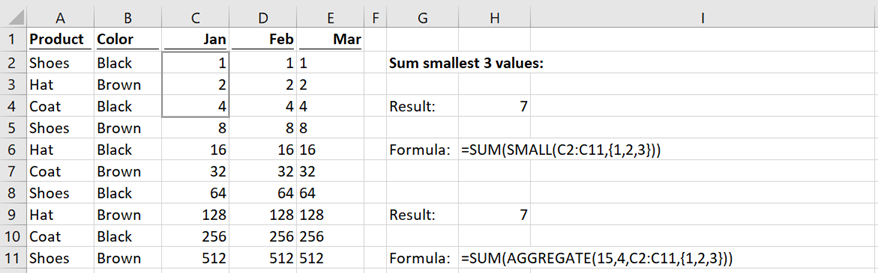The formula in Cell H4 is:

`{=SUM(SMALL(C2:C11,{1,2,3}))}`

This array formula will sum the smallest three values.

The formula in Cell H9 is:

`=SUM(AGGREGATE(15,4,C2:C11,{1,2,3}))`

AGGREGATE can also be used as an equivalent to the SMALL function.

## SUM every n rows

This final example now gets a bit more complicated. This will SUM every third cell, but could be adapted to sum every 4th, 5th, or nth row.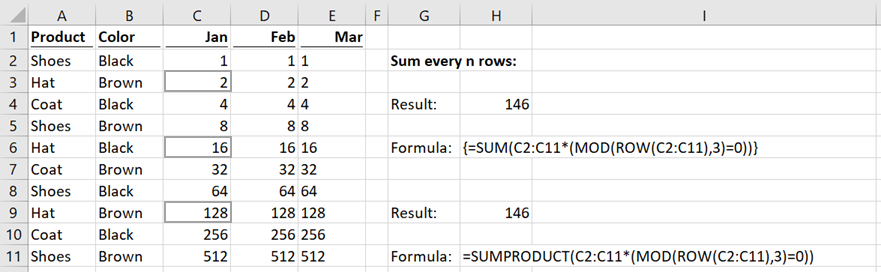The formula in Cell H4 is:

`=SUM(C2:C11*(MOD(ROW(C2:C11),3)=0))`

This formula will take some explaining, which is outside the scope of this article. But trust me, it works.

The formula in Cell H9 is:

`=SUMPRODUCT(C2:C11*(MOD(ROW(C2:C11),3)=0))`

Once again, we use SUMPRODUCT to avoid the array function.

## Conclusion

You’ve just seen ten examples of new ways to use the SUM function. Whilst you might not use SUM in this way, we have covered a lot of Excel techniques in this post, such as array formulas, boolean logic and the intersection operator to name a few. So, go and experiment.  How can you use these techniques to master Excel?

Happy spreadsheet day… enjoy 🙂